Share

Books Shortlist
Your shortlist is empty

# NCERT solutions for Class 10 Mathematics chapter 13 - Surface Areas and Volumes

## Mathematics Textbook for Class 10

#### NCERT Mathematics Class 10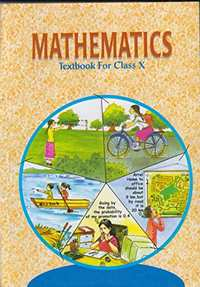## Chapter 13: Surface Areas and Volumes

Ex. 13.10Ex. 13.20Ex. 13.30Ex. 13.40

#### Chapter 13: Surface Areas and Volumes Exercise 13.10 solutions [Pages 244 - 245]

Ex. 13.10 | Q 1 | Page 244

2 cubes each of volume 64 cm3 are joined end to end. Find the surface area of the resulting cuboid.

Ex. 13.10 | Q 2 | Page 244

A vessel is in the form of a hollow hemisphere mounted by a hollow cylinder. The diameter of the hemisphere is 14 cm and the total height of the vessel is 13 cm. Find the inner surface area of the vessel. [Use π = 22/7]

Ex. 13.10 | Q 3 | Page 244

A toy is in the form of a cone of radius 3.5 cm mounted on a hemisphere of same radius. The total height of the toy is 15.5 cm. Find the total surface area of the toy [Use π =22/7]

Ex. 13.10 | Q 4 | Page 244

A cubical block of side 7 cm is surmounted by a hemisphere. What is the greatest diameter the hemisphere can have? Find the surface area of the solid. [Use π = 22/7]

Ex. 13.10 | Q 5 | Page 244

A hemispherical depression is cut out from one face of a cubical wooden block such that the diameter l of the hemisphere is equal to the edge of the cube. Determine the surface area of the remaining solid.

Ex. 13.10 | Q 6 | Page 244

A medicine capsule is in the shape of cylinder with two hemispheres stuck to each of its ends (see the given figure). The length of the entire capsule is 14 mm and the diameter of the capsule is 5 mm. Find its surface area. [Use π = 22/7]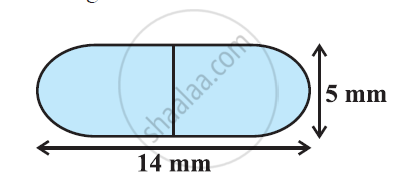Ex. 13.10 | Q 7 | Page 245

A tent is in the shape of a cylinder surmounted by a conical top. If the height and diameter of the cylindrical part are 2.1 m and 4 m respectively, and the slant height of the top is 2.8 m, find the area of the canvas used for making the tent. Also, find the cost of the canvas of the tent at the rate of Rs 500 per m2. (Note that the base of the tent will not be covered with canvas.) [Use π=22/7]

Ex. 13.10 | Q 8 | Page 245

From a solid cylinder whose height is 2.4 cm and diameter 1.4 cm, a conical cavity of the same height and same diameter is hollowed out. Find the total surface area of the remaining solid to the nearest cm[Use π = 22/7]

Ex. 13.10 | Q 9 | Page 245

A wooden article was made by scooping out a hemisphere from each end of a solid cylinder, as shown in given figure. If the height of the cylinder is 10 cm, and its base is of radius 3.5 cm, find the total surface area of the article [Use π = 22/7]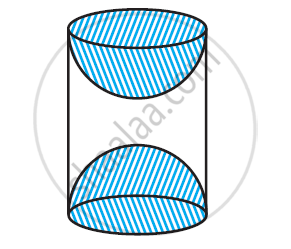#### Chapter 13: Surface Areas and Volumes Exercise 13.20 solutions [Pages 247 - 248]

Ex. 13.20 | Q 1 | Page 247

A solid is in the shape of a cone standing on a hemisphere with both their radii being equal to 1 cm and the height of the cone is equal to its radius. Find the volume of the solid in terms of π.

Ex. 13.20 | Q 2 | Page 247

Rachel, an engineering student, was asked to make a model shaped like a cylinder with two cones attached at its two ends by using a thin aluminum sheet. The diameter of the model is 3 cm and its length is 12 cm. if each cone has a height of 2 cm, find the volume of air contained in the model that Rachel made. (Assume the outer and inner dimensions of the model to be nearly the same.)  Use [Π = 22/7]

Ex. 13.20 | Q 3 | Page 248

A gulab jamun, contains sugar syrup up to about 30% of its volume. Find approximately how much syrup would be found in 45 gulab jamuns, each shaped like a cylinder with two hemispherical ends with length 5 cm and diameter 2.8 cm (see the given figure).  Use [Π = 22/7]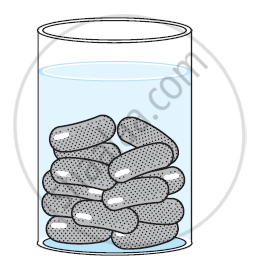Ex. 13.20 | Q 4 | Page 248

A pen stand made of wood is in the shape of a cuboid with four conical depressions to hold pens. The dimensions of the cuboids are 15 cm by 10 cm by 3.5 cm. The radius of each of the depressions is 0.5 cm and the depth is 1.4 cm. Find the volume of wood in the entire stand (see the following figure).  Use [Π = 22/7]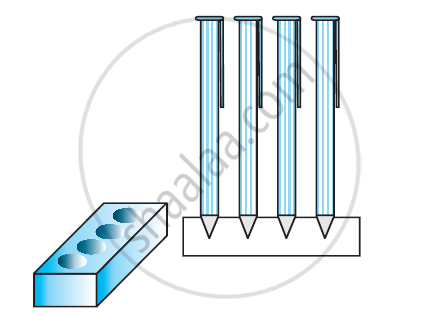Ex. 13.20 | Q 5 | Page 248

A vessel is in the form of an inverted cone. Its height is 8 cm and the radius of its top, which is open, is 5 cm. It is filled with water up to the brim. When lead shots, each of which is a sphere of radius 0.5 cm are dropped into the vessel, one-fourth of the water flows out. Find the number of lead shots dropped in the vessel.

Ex. 13.20 | Q 6 | Page 248

A solid iron pole consists of a cylinder of height 220 cm and base diameter 24 cm, which is surmounted by another cylinder of height 60 cm and radius 8 cm. Find the mass of the pole, given that 1 cm3 of iron has approximately 8 g mass. [Use π = 3.14]

Ex. 13.20 | Q 7 | Page 248

A solid consisting of a right circular cone of height 120 cm and radius 60 cm standing on a hemisphere of radius 60 cm is placed upright in a right circular cylinder full of water such that it touches the bottom. Find the volume of water left in the cylinder, if the radius of the cylinder is 60 cm and its height is 180 cm. Use [Π = 22/7]

Ex. 13.20 | Q 8 | Page 248

A spherical glass vessel has a cylindrical neck 8 cm long, 2 cm in diameter; the diameter o the spherical part is 8.5 cm. By measuring the amount of water it holds, a child finds its volume to be 345 cm3. Check whether she is correct, taking the above as the inside measurements, and π = 3.14

#### Chapter 13: Surface Areas and Volumes Exercise 13.30 solutions [Pages 251 - 252]

Ex. 13.30 | Q 1 | Page 251

A metallic sphere of radius 4.2 cm is melted and recast into the shape of a cylinder of radius 6 cm. Find the height of the cylinder.

Ex. 13.30 | Q 2 | Page 251

Metallic spheres of radii 6 cm, 8 cm, and 10 cm, respectively, are melted to form a single solid sphere. Find the radius of the resulting sphere.

Ex. 13.30 | Q 3 | Page 251

A 20 m deep well with diameter 7 m is dug and the earth from digging is evenly spread out to form a platform 22 m by 14 m. Find the height of the platform. [Use Π = 22/7]

Ex. 13.30 | Q 4 | Page 251

A well of diameter 3 m is dug 14 m deep. The earth taken out of it has been spread evenly all around it in the shape of a circular ring of width 4 m to form an embankment. Find the height of the embankment.

Ex. 13.30 | Q 5 | Page 251

A container shaped like a right circular cylinder having diameter 12 cm and height 15 cm is full of ice cream. The ice cream is to be filled into cones of height 12 cm and diameter 6 cm, having a hemispherical shape on the top. Find the number of such cones which can be filled with ice cream.

Ex. 13.30 | Q 6 | Page 251

How many silver coins, 1.75 cm in diameter and of thickness 2 mm, must be melted to form a cuboid of dimensions 5.5 cm × 10 cm × 3.5 cm? [Use  π=22/7]

Ex. 13.30 | Q 7 | Page 252

A cylindrical bucket, 32 cm high and with radius of base 18 cm, is filled with sand. This bucket is emptied on the ground and a conical heap of sand is formed. If the height of the conical heap is 24 cm. Find the radius and slant height of the heap.

Ex. 13.30 | Q 8 | Page 252

Water in canal, 6 m wide and 1.5 m deep, is flowing with a speed of 10 km/h. how much area will it irrigate in 30 minutes, if 8 cm of standing water is needed?

Ex. 13.30 | Q 9 | Page 252

A farmer connects a pipe of internal diameter 20 cm form a canal into a cylindrical tank in her field, which is 10 m in diameter and 2 m deep. If water flows through the pipe at the rate of 3 km/h, in how much time will the tank be filled?

#### Chapter 13: Surface Areas and Volumes Exercise 13.40 solutions [Page 257]

Ex. 13.40 | Q 1 | Page 257

A drinking glass is in the shape of a frustum of a cone of height 14 cm. The diameters of its two circular ends are 4 cm and 2 cm. Find the capacity of the glass.  [use π=22/7]

Ex. 13.40 | Q 2 | Page 257

The slant height of a frustum of a cone is 4 cm and the perimeters (circumference) of its circular ends are 18 cm and 6 cm. find the curved surface area of the frustum.

Ex. 13.40 | Q 3 | Page 257

fez, the cap used by the Turks, is shaped like the frustum of a cone (see the figure given below). If its radius on the open side is 10 cm, radius at the upper base is 4 cm and its slant height is 15 cm, find the area of material use for making it.  [use π=22/7]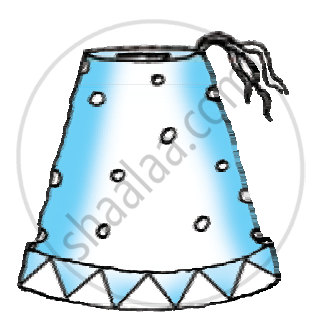Ex. 13.40 | Q 4 | Page 257

A container, opened from the top and made up of a metal sheet, is in the form of a frustum of a cone of height 16 cm with radii of its lower and upper ends as 8 cm and 20 cm respectively. Find the cost of the milk which can completely fill the container, at the rate of Rs.20 per litre. Also find the cost of metal sheet used to make the container, if it costs Rs.8 per 100 cm2. [Take π = 3.14]

Ex. 13.40 | Q 5 | Page 257

A metallic right circular cone 20 cm high and whose vertical angle is 60° is cut into two parts at the middle of its height by a plane parallel to its base. If the frustum so obtained is drawn into a wire of diameter 1/16 cm, find the length of the wire [use π=22/7]

#### Chapter 13: Surface Areas and Volumes Exercise 13.40 solutions [Page 258]

Ex. 13.40 | Q 1 | Page 258

A copper wire, 3 mm in diameter, is wound about a cylinder whose length is 12 cm, and diameter 10 cm, so as to cover the curved surface of the cylinder. Find the length and mass of the wire, assuming the density of copper to be 8.88 g per cm3.

Ex. 13.40 | Q 2 | Page 258

A right triangle whose sides are 3 cm and 4 cm (other than hypotenuse) is made to revolve about its hypotenuse. Find the volume and surface area of the double cone so formed. (Choose value of π as found appropriate.)

Ex. 13.40 | Q 3 | Page 258

A cistern, internally measuring 150 cm × 120 cm × 110 cm, has 129600 cm3 of water in it. Porous bricks are placed in the water until the cistern is full to the brim. Each brick absorbs one-seventeenth of its own volume of water. How many bricks can be put in without overflowing the water, each brick being 22.5 cm × 7.5 cm × 6.5 cm?

Ex. 13.40 | Q 4 | Page 258

In one fortnight of a given month, there was a rainfall of 10 cm in a river valley. If the area of the valley is 7280 km2, show that the total rainfall was approximately equivalent to the addition to the normal water of three rivers each 1072 km long, 75 m wide and 3 m deep.

Ex. 13.40 | Q 5 | Page 258

An oil funnel made of tin sheet consists of a 10 cm long cylindrical portion attached to a frustum of a cone. If the total height is 22 cm, diameter of the cylindrical portion is 8 cm and the diameter of the top of the funnel is 18 cm, find the area of the tin sheet required to make the funnel (see the given figure).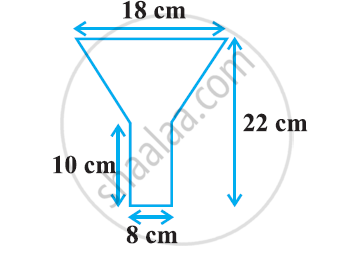Ex. 13.40 | Q 6 | Page 258

Derive the formula for the curved surface area and total surface area of the frustum of cone.

Ex. 13.40 | Q 7 | Page 258

Derive the formula for the volume of the frustum of a cone.

## Chapter 13: Surface Areas and Volumes

Ex. 13.10Ex. 13.20Ex. 13.30Ex. 13.40

#### NCERT Mathematics Class 10## NCERT solutions for Class 10 Mathematics chapter 13 - Surface Areas and Volumes

NCERT solutions for Class 10 Maths chapter 13 (Surface Areas and Volumes) include all questions with solution and detail explanation. This will clear students doubts about any question and improve application skills while preparing for board exams. The detailed, step-by-step solutions will help you understand the concepts better and clear your confusions, if any. Shaalaa.com has the CBSE Mathematics Textbook for Class 10 solutions in a manner that help students grasp basic concepts better and faster.

Further, we at Shaalaa.com are providing such solutions so that students can prepare for written exams. NCERT textbook solutions can be a core help for self-study and acts as a perfect self-help guidance for students.

Concepts covered in Class 10 Mathematics chapter 13 Surface Areas and Volumes are Volume of a Combination of Solids, Surface Areas and Volumes Examples and Solutions, Introduction of Surface Areas and Volumes, Frustum of a Cone, Conversion of Solid from One Shape to Another, Surface Area of a Combination of Solids.

Using NCERT Class 10 solutions Surface Areas and Volumes exercise by students are an easy way to prepare for the exams, as they involve solutions arranged chapter-wise also page wise. The questions involved in NCERT Solutions are important questions that can be asked in the final exam. Maximum students of CBSE Class 10 prefer NCERT Textbook Solutions to score more in exam.

Get the free view of chapter 13 Surface Areas and Volumes Class 10 extra questions for Maths and can use Shaalaa.com to keep it handy for your exam preparation

S